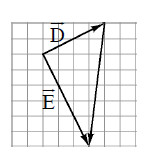### Home > CALC > Chapter 9 > Lesson 9.3.2 > Problem9-104

9-104.

On the same resource page, draw $\vec { A } - \vec { B }$  and $\vec { B } - \vec { A }$.

1. What one word describes the relationship between these vectors?

How is $5-3$ related to $3-5$?

2. Draw $\vec { D }$ and $\vec { E }$ with a third vector from the head of $\vec { D }$ to the head of $\vec { E }$ as shown above. Label the third vector using $\vec { D }$ and $\vec { E }$.

Look at the diagram. Switch the initial and end points of vector D.